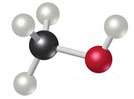# Problem: Calculate the percentage of carbon by mass in each of the compounds represented by the following models. (Carbon atoms are black spheres, hydrogen are grey, oxygen are red.)

###### FREE Expert Solution

Mass percent:

Compound is: CH3OH

• Molar mass CH3OH = 32.04 g/mol
81% (163 ratings)###### Problem Details

Calculate the percentage of carbon by mass in each of the compounds represented by the following models. (Carbon atoms are black spheres, hydrogen are grey, oxygen are red.)Frequently Asked Questions

What scientific concept do you need to know in order to solve this problem?

Our tutors have indicated that to solve this problem you will need to apply the Mass Percent concept. You can view video lessons to learn Mass Percent. Or if you need more Mass Percent practice, you can also practice Mass Percent practice problems.